# 原理化毛发 BSDF节点¶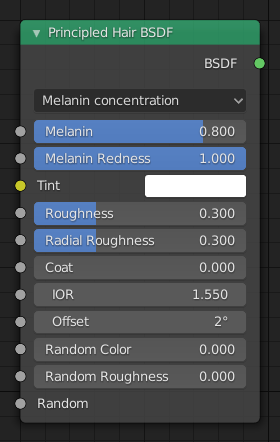Melanin concentration选项下的原理化毛发 BSDF节点界面。

Tip

## 输入选项¶

Hint

$\sigma_{a} = \frac{\ln(Color)} {\left(5.969 - 0.215\beta_{N} + 2.532\beta_{N}^{2} - 10.73\beta_{N}^{3} + 5.574\beta_{N}^{4} + 0.245\beta_{N}^{5}\right)^{2}}$Hint

$melanin\_qty = -\ln(\max(1.0 - Melanin, 0.0001))$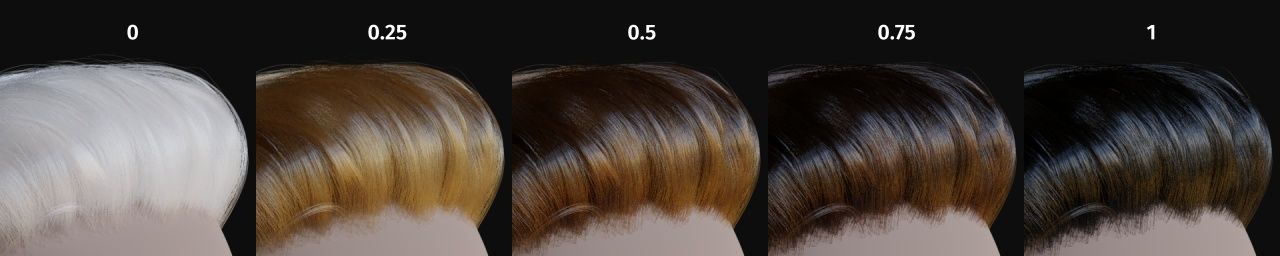Hint

$\begin{split}\sigma_{a} = eumelanin * \left[\begin{matrix} 0.506 \\ 0.841 \\ 1.653 \\ \end{matrix}\right] + pheomelanin * \left[\begin{matrix} 0.343 \\ 0.733 \\ 1.924 \\ \end{matrix}\right]\end{split}$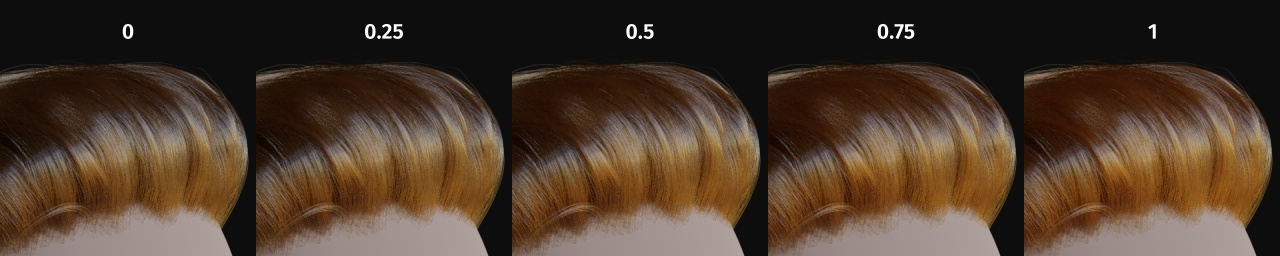Hint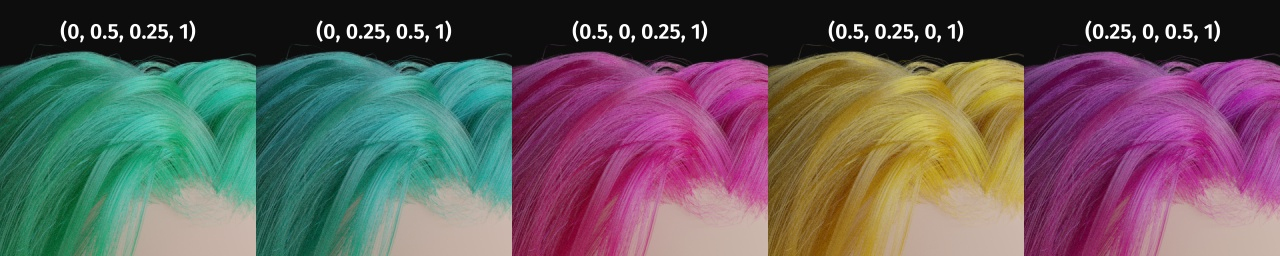Absorption Coefficient

Specify how much the glints are smoothed in the direction of the hair shaft. Too low values will smoothen the hair to the point of looking almost metallic, making glints look like Fireflies; while setting it too high will result in a Lambertian look.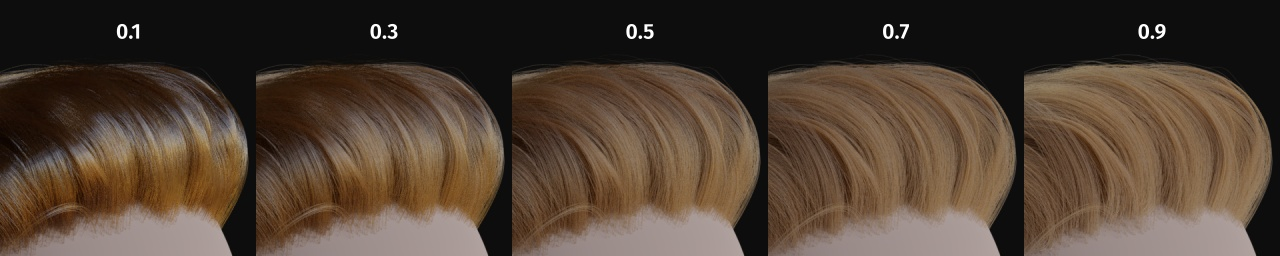Hint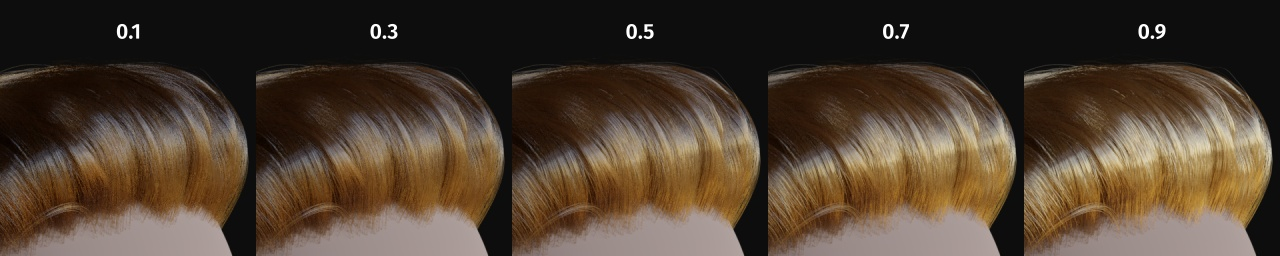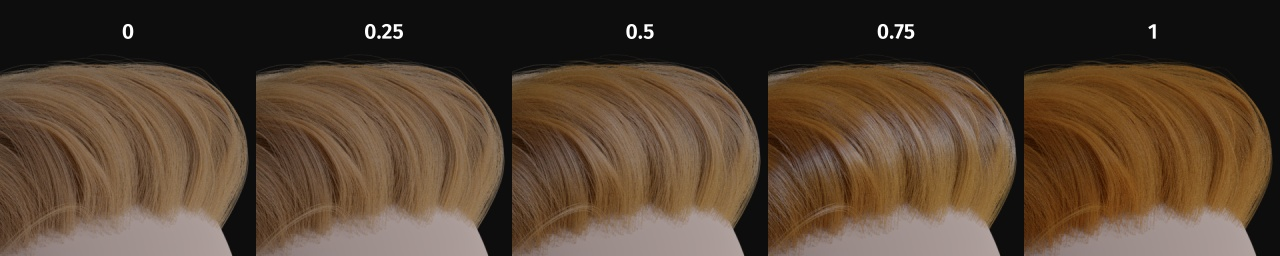IOR 折射率

HintHint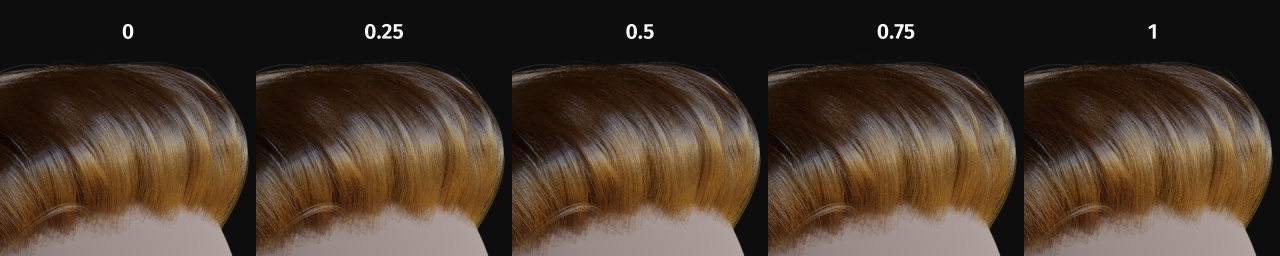## 属性¶

Color Parametrization

Direct Coloring

Melanin Concentration

• White (Melanin $$0$$)

• 亚麻色 (黑色素值设为 $$0.25$$)

• 淡红色 (黑色素值设为 $$0.5$$)

• 棕色 (黑色素值设为 $$0.75$$)

• 黑色 (黑色素值设为 $$1$$)

Absorption Coefficient

BSDF